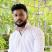# Optics: Overview, Questions, Preparation

Optics 2021 ( Optics )

1.1K ViewsRajdeep DasAssistant Manager- Content

Updated on Jun 24, 2021 11:30 IST
1. What is the Lens formula?
2. Derivation of lens formula
3. Derivation of lens formula for class 12
4. Illustrated examples
5. FAQs on Derivation of lens formula

## What is the Lens formula?

The lens formula can be described as the relationship between the focal length, the distance of an object, and the lens’ image. The lens formula is appropriate for both convex and concave lenses. The thickness of the lenses is negligible. The lens formula is as follows:

1/v – 1/v = 1/f

Here, v is the distance of an image

f is the focal length

u is the distance of an object.

## Derivation of lens formula

There are two types of lens, concave and convex lens.

Derivation on the convex lens:

Let us consider a convex lens having an optical center as O, F being the principal focus.

So, A’B’/AB = OB’/OB

The triangles ΔABO and ΔA’B’O are similar to each other

In the same way, ΔA’B’F and ΔOCF are similar to each other

So, A’B’/OC = FB’/OF

Now, OC = AB

So, A’B’/AB = FB’/OF

From the above equations, we can get,

OB’/OB = FB’/OF = (OB’ – OF) / OF

Using sign convention,

OB = -u, OB’ = v and OF = f

We get, v/-u = (v-f) / f

Which means vf = -uv + uf

Or, uv = uf – vf

Hence, 1/f = 1/v + 1/u

Derivation on the concave lens:

Let us assume that the AB is perpendicular to the principal axis and the distance is f of the convex lens. Here, we can assume,

OF1 as focal length

OA as the distance of the object

OA as image distance,

And ΔOAB and ΔOA’B’ are equal to each other

Angle BAO = angle B’A’O = 90°, and O is common in both the angles.

So, angle AOB = angle A’OB’

Now, angle. ABO and A’B’O are equal

And, A’B’/AB = OA’/OA

So, A’B’/OC = A’F1/ OF1

Now from the above equations, we get,

OA’ / OA = (OF1 – OA’) OF1

-v / -u = (-f - - v) / -f

V / u = (-f + v) / -f

-vf = -uf +uv

Let us now divide the above equation by uvf

-1/ u = -1/v + 1/f

Hence, 1/f = 1/v – 1/u

And this is the formula of the concave lens.

## Derivation of lens formula for class 12

As per the new pattern 2021, the chapter ' Optics' holds a significant weightage of 7 marks. These 7 marks include 2 questions in total, one short question of 2 marks, and one long question of 5 marks.

## Illustrated examples

Example 1) Illustrate the lens formula for the convex lens.

Answer – The lens formula for convex lens is 1/f = 1/v + 1/u

Example 2) Write the sign of v in a concave lens.

Answer – The sign of v in the concave lens is positive due to the incident rays.

Example 3) Write the name of the lens used in the torchlight.

Answer – Torch lights use a diverging lens, also known as a concave lens to make the parallel light spread out evenly.

## FAQs on Derivation of lens formula

1) What is the lens formula?

Answer - The lens formula can be described as the relationship between the focal length, a distance of an object, and the distance of an image of the lens.

2) What is the SI unit of the lens?

Answer – The SI unit of the lens is Dioptre or diopter. 1 dioptre is equal to 1 m -1 .

3) What is the difference between mirror and lens?

Answer – The mirror can be straight or round in shape and can be formed into different shapes whereas lenses are curved from the surfaces

4) What lens or mirrors are used by the dentists?

Answer – Dentists use the concave mirror to get magnified and clear reflection of the mouth. They refract the light to see the zoomed-in image.

5) What lenses are used in the street lights?

Answer – Street lights use convex mirrors because the convex mirror reflects the light, and it spreads it in the broader area.